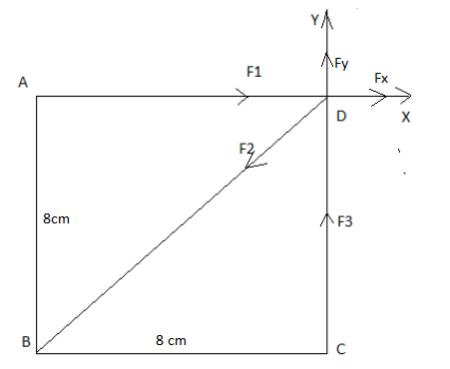# Three charges, each of magnitude 7 nC, are at separate corners of a square of edge length 8 cm....

## Question:

Three charges, each of magnitude 7 nC, are at separate corners of a square of edge length 8 cm. The two charges at opposite corners are positive, and the other charge is negative. Find the force exerted by these charges on a fourth charge q = +3 nC at the remaining (upper right) corner. (Assume the +x axis is directed to the right and the +y axis is directed upward.)

1) N i

2) N j

## Electrostatic Force.

The electrostatic force is repulsive if the interacting charges are of the same polarity and attractive if the interacting charges are of opposite polarity.

Nature of the charge of anybody is of any of three types positive, negative and neutral.

Electrostatic force follows inverse square law and it has its range to infinity.

Electrostatic Force at any a charge {eq}q {/eq} at a distance {eq}r {/eq} due to a charge {eq}Q {/eq} is {eq}\dfrac{\kappa \times q\times Q}{r^2}\ N {/eq}

• {eq}\kappa \ =\ 8.98\times 10^{9}\ \dfrac{N\cdot m^2}{C^2} {/eq}• {eq}q_1 {/eq} = Charge at point A = {eq}7\ nC {/eq}
• {eq}q_2 {/eq} = Charge at point B = {eq}-\ 7\ nC {/eq}
• {eq}q_3 {/eq} = Charge at point C = {eq}7\ nC {/eq}
• {eq}q_4 {/eq} = Charge at point D = {eq}+\ 3\ nC {/eq}
• {eq}a {/eq} = side of square = {eq}8\ cm\ =\ 0.08\ m {/eq}
• {eq}F_1 {/eq} = magnitude of repulsive electrostatic force on charge {eq}q_4 {/eq} due to charge {eq}q_1 {/eq} in X-axis.
• {eq}F_2 {/eq} = magnitude of attractive electrostatic force on charge {eq}q_4 {/eq} due to charge {eq}q_2 {/eq} in the direction {eq}135^\circ {/eq} clockwise to X-axis.
• {eq}F_3 {/eq} = magnitude of repulsive electrostatic force on charge {eq}q_4 {/eq} due to charge {eq}q_4 {/eq} in Y-axis.
• {eq}F_x {/eq} = Net force in X-axis.
• {eq}F_y {/eq} = Net force in Y-axis.

We know that,

Electrostatic Force at any a charge {eq}q {/eq} at a distance {eq}r {/eq} due to a charge {eq}Q {/eq} is {eq}\dfrac{\kappa \times q\times Q}{r^2}\ N {/eq}

• {eq}\kappa \ =\ 8.98\times 10^{9}\ \dfrac{N\cdot m^2}{C^2} {/eq}

Part A

Now,

Magnitude of net force in X-axis is

{eq}\begin{align} F_x\ &=\ F_1\ -\ F_2\times \cos 45^\circ\\ &=\ \dfrac{\kappa \times q_3\times q_4}{a^2}\ +\ \dfrac{\kappa \times q_4\times q_2}{\left ( \sqrt 2a \right )^2}\times \cos 45^\circ \\ &=\ \dfrac{ 8.98\times 10^{9}\times 7\times 10^{-9}\times 3\times 10^{-9}}{0.08^2}\ -\ \dfrac{ 8.98\times 10^{9}\times 7\times 10^{-9}\times 3\times 10^{-9}}{\left ( \sqrt 2\times 0.08 \right )^2}\times \dfrac{1}{\sqrt 2} \\ &=\ 1.904795\times 10^{-5}\ N \end{align} {/eq}

Hence, Net force in X-axis is {eq}1.904795\times 10^{-5}\ N \ i {/eq}

Part B

Magnitude of net force in Y-axis is

{eq}\begin{align} F_y\ &=\ F_3\ -\ F_2\times \sin 45^\circ &=\ \dfrac{\kappa \times q_4\times q_1}{a^2}\ -\ \dfrac{\kappa \times q_4\times q_2}{\left ( \sqrt 2a \right )^2}\times \sin 45^\circ \ &=\ \dfrac{8.98\times 10^{9}\times 7\times 10^{-9}\times 3\times 10^{-9}}{0.07^2} \ -\ \dfrac{8.98\times 10^{9}\times 7\times 10^{-9}\times 3\times 10^{-9}}{2\times 0.07^2}\times \dfrac{1}{\sqrt2} &=\ 1.904795\times 10^{-5}\ N \end{align} {/eq}

Hence, Hence, Net force in Y-axis is {eq}1.904795\times 10^{-5}\ N \ j {/eq}Coulomb's Law: Variables Affecting the Force Between Two Charged Particles

from

Chapter 6 / Lesson 3
39K

In the 18th century, Charles Coulomb uncovered the secrets of electrostatic force between charged particles. The results of his experiments led to what is now known as Coulomb's Law, which tells us how force, charge, and distance are all related.